### Home > CC1 > Chapter Ch6 > Lesson 6.2.4 > Problem6-106

6-106.

Find the perimeter and area of each figure made of algebra tiles below. Homework Help ✎

1.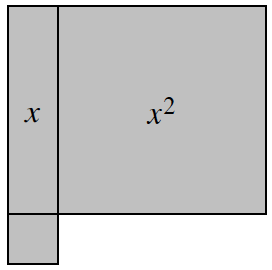Remember, the small square is a unit tile, which is a 1 × 1 square.

The dimensions have been placed on the algebra tiles for you.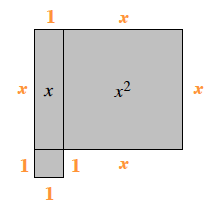4x + 4 units

1.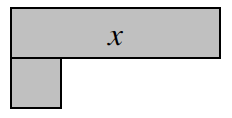Looking at the algebra tiles below, do you understand why the length of one side is labeled x − 1?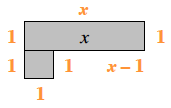Since part of the side with length x touches the unit tile, the side that is included in the calculation of the perimeter is x − 1.

1.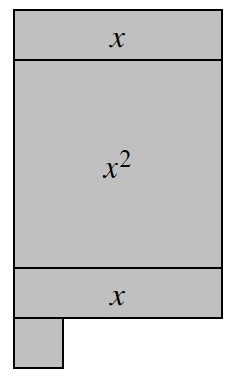Refer to parts (a) and (b).